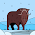### Matplotlib with a dark background

The Matplotlib package for the Python language is pretty powerful, but sometimes it can be a bit frustrating to use. Some things simply aren't intuitive or obvious and the documentation can be a bit obtuse at times.

This time, I was trying to get Matplotlib to plot in negative -- not the usual black-on-white, by white-on-black. This can be useful for websites... especially those when you want to plot data on the same page as faint aurora images. While I found changing the axis face colour pretty quickly, the borders remained stubbornly white. I did figure it out in the end, but it took a while. Here's how to do it.

```#!/usr/bin/env python

import matplotlib.pyplot as plt

# Create some data
x = [0.0, 1.0, 2.0, 3.0]
y = [2.0, 0.0, 1.0, 7.0]

# Create a new figure, and set the style
fig=plt.figure()
plt.style.use('dark_background')
```
```# Plot the data and set the labels.
plt.plot(x,y,color='r')
plt.xlabel("x")
plt.ylabel("y")

# Save the figure to a file
plt.savefig("dark.png")```

This results in a plot that looks like this:

The axes face (the region within the box) and the figure face (the rest of the "page") are thus black and all the labels, etc. are set to white.

Actually, you can control all the colours of the plot, including the axes and figure face colours. To do this, you need to set the relevant rcParams. There are actually lots of these and they can be used to control most of the aspects of the way Matplotlib plots.

For example, I'll add two more lines to the previous example, which gives:

```#!/usr/bin/env python
import matplotlib.pyplot as plt

# Create some data
x = [0.0, 1.0, 2.0, 3.0]
y = [2.0, 0.0, 1.0, 7.0]

# Create a new figure, and set the style
fig=plt.figure()
plt.style.use('dark_background')
plt.rcParams['axes.facecolor'] = 'g'
plt.rcParams['savefig.facecolor'] = 'b'

# Plot the data and set the labels.
plt.plot(x,y,color='r')
plt.xlabel("x")
plt.ylabel("y")

# Save the figure to a file
plt.savefig("face.png")

```

Resulting in this plot:

Important: note that the rcParam to change is "savefig.facecolor", not "figure.facecolor"!

1.Great tip. It worth mentioning that to go back to the default style sheet you may use: plt.style.use('default').

2.3.Doing animations for space physics in matplotlib is SO much nicer with black background and no axis :3 thumbs up